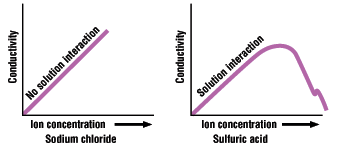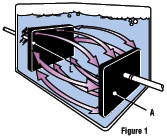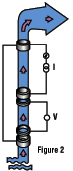Conductivity Theory and Technical Tips

Conductivity

Definition of Conductivity    Units of Measurement     Conductivity Meter Calibration and Cell Maintenance    Conductivity Temperature Compensation    Conductivity Cells    Important Features to Consider

Definition of Conductivity

Conductivity is the ability of a material to conduct electric current. The principle by which instruments measure conductivity is simpletwo plates are placed in the sample, a potential is applied across the plates (normally a sine wave voltage), and the current that passes through the solution is measured. Conductivity (G), the inverse of resistivity (R), is determined from the voltage and current values according to Ohm's law.

 G = 1  R = I (amps) E (volts)

Since the charge on ions in solution facillitates the conductance of electrical current, the conductivity of a solution is proportional to its ion concentration.In some situations, however, conductivity may not correlate directly to concentration. The graphs shown here illustrate the relationship between conductivity and ion concentration for two common solutions. Notice that the graph is linear for sodium chloride solution, but not for highly concentrated sulfuric acid. Ionic interactions can alter the linear relationship between conductivity and concentration in some highly concentrated solutions.

Units of MeasurementThe basic unit of conductance is the siemen (S), formerly called the mho. Since cell geometry affects conductivity values, standardized measurements are expressed in specific conductivity units (S/cm) to compensate for variations in electrode dimensions. Specific conductivity (C) is simply the product of measured conductivity (G) and the electrode cell constant (L/A), where L is the length of the column of liquid between the electrodes and A is the area of the electrodes (see illustration).

C = G x (L/A)

If the cell constant(K) is 1 cm-1, the specific conductivity is the same as the measured conductivity of the solution. If other cell constants are used, most meters will automatically compensate for the change in cell geometry. To save room, cm-1 is not shown when cell constants are listed.

Although we specify conductivity ranges for our products in µS or mS, due to space limitations these ranges should be understood to reflect specific conductivity in µS/cm or mS/cm, respectively.

1 µS/cm = 0.001 mS/cm = 0.000001 S/cm = 1 µmho/cm

The following table shows optimum conductivity ranges for cells of three different constants:

 CellConstant(K) Optimum Conductivity Range(µS/cm) 0.1 0.5 to 400 1.0 10 to 2000 10.0 1000 to 200,000

Conductivity Meter Calibration and Cell Maintenance

Conductivity meters and cells should be calibrated to a standard solution before using. Select a standard that is closest to the conductivity of the solution to be measured. Polarized or fouled electrodes must be replatinized or cleaned to renew active surface of the cell. In most situations, hot water with a mild liquid detergent is an effective cleanser. Acetone easily cleans most organic matter, and chlorous solutions will remove algae, bacteria, or molds. Do not use abrasives to clean an electrode. Replace this cell if all else fails.

The conductivity of some common solutions is shown in the table below.

 Solution Conductivity Pure water 0.055 µS/cm Power plant boiler water 1.0 µS/cm Good city water 50 µS/cm Ocean water 53 mS/cm 31.0% HNO3 865 mS/cm

Conversions:

 Multiply → to get to get ← Divide µS/cm 1 µmho/cm mS/cm 1000 µS/cm µS/cm 0.5 ppm

Conductivity Temperature Compensation

Conductivity measurements are temperature dependent. The degree to which temperature affects conductivity varies from solution to solution and can be calculated using the following formula:

Gt = Gtcal{1 α(t-tcal)}

where:
Gt = conductivity at any temperature t in °C
Gtcal = conductivity at calibration temperature tcal in °C
α = temperature coefficient of solution at tcal in °C

Common alphas (α) are listed in the table below. To determine the α of other solutions, simply measure conductivity at a range of temperatures and graph the change in conductivity versus the change in temperature. Divide the slope of the graph by Gtcal to get α.

 Substanceat 25°C Concentration Alpha (α) HCl 10 wt% 1.56 KCl 10 wt% 1.88 H2SO4 50 wt% 1.93 NaCl 10 wt% 2.14 HF 1.5 wt% 7.20 HNO3 31 wt% 31.0

All meters in our catalog have either fixed or adjustable automatic temperature compensation referenced to a standard temperatureusually 25°C. Most meters with fixed temperature compensation use an a of 2% per °C (the approximate a of NaCl solutions at 25°C). Meters with adjustable temperature compensation let you adjust the alpha factor to more closely match the alpha factor of your solution.

Conductivity CellsMost conductivity meters have a two-electrode cell (see illustration) available in either dip or flow-through styles. The electrode surface is usually platinum, titanium, gold-plated nickel, or graphite.

Four-electrode cells use a reference voltage to compensate for any polarization or fouling of the electrode plates. The reference voltage ensures that measurements indicate actual conductivity independent of electrode condition, resulting in higher accuracy for measuring over wide ranges.

Important Features to Consider
• Autoranging: Meter automatically selects the appropriate range for measurement. There is no need to change the dial, multiply values on the display, turn a potentiometer, or manually select a range.
• Temperature compensation: A cell with built-in temperature sensor allows the meter to make adjustments to the conductivity or TDS readings based on changes in solution temperature.
• TDS conversion factor: When a solution does not have a similar ionic content to natural water or salt water, then a TDS conversion factor is needed to automatically adjust the readings.
• Adjustable temperature coefficients: The TDS of certain samples, such as alcohols and pure water, are affected by changes in temperature. An adjustable temperature coefficient allows the user to compensate for temperature changes on the solution being measured.
• Adjustable cell constant: Adjusts the reading on the display to reflect use of a cell with a constant other than K=1.

Go to Top